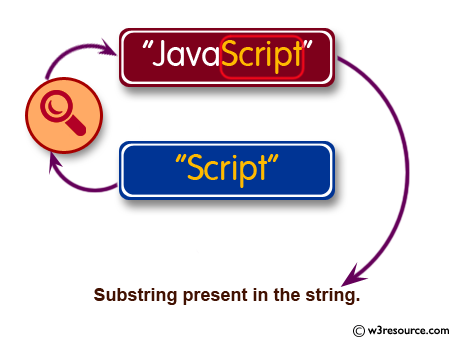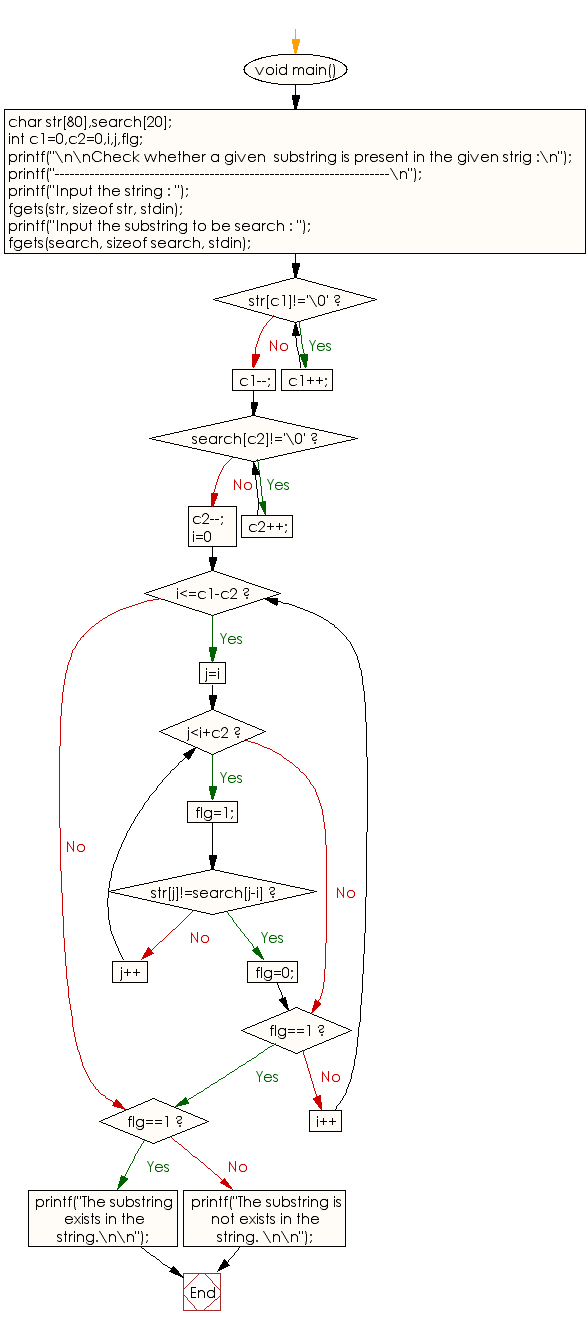﻿ C exercises: Check whether a given substring is present in the given string - w3resource# C Programming: Check whether a given substring is present in the given string

## C String: Exercise-14 with Solution

Write a C program to check whether a given substring is present in the given string.Sample Solution:

C Code:

``````#include <stdio.h>
void main()
{
char str,search;
int c1=0,c2=0,i,j,flg;

printf("\n\nCheck whether a given  substring is present in the given string :\n");
printf("-------------------------------------------------------------------\n");

printf("Input the string : ");
fgets(str, sizeof str, stdin);

printf("Input the substring to be search : ");
fgets(search, sizeof search, stdin);

while (str[c1]!='\0')
c1++;
c1--;

while (search[c2]!='\0')
c2++;
c2--;

for(i=0;i<=c1-c2;i++)
{
for(j=i;j<i+c2;j++)
{
flg=1;
if (str[j]!=search[j-i])
{
flg=0;
break;
}
}
if (flg==1)
break;
}
if (flg==1)
printf("The substring exists in the string.\n\n");
else
printf("The substring is not exists in the string. \n\n");
}
```
```

Sample Output:

```Check whether a given  substring is present in the given string :
-------------------------------------------------------------------
Input the string : This is a test string.
Input the substring to be search : search
The substring is not exists in the string.
```

Flowchart :C Programming Code Editor:

Improve this sample solution and post your code through Disqus.

What is the difficulty level of this exercise?

﻿